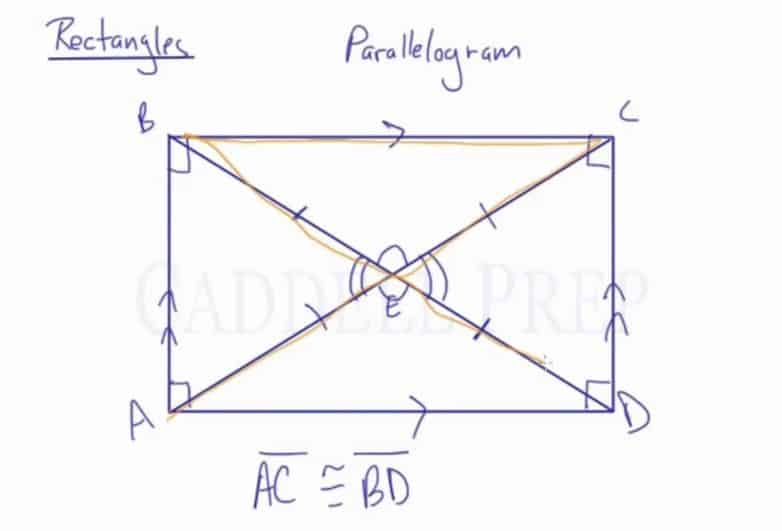Learn about the properties of rectangles including relationships among opposite sides, opposite angles, adjacent angles, diagonals and angles formed by diagonals.

Rectangle: A parallelogram with a right angle.

Rectangles are similar to parallelograms, so opposite sides are congruent and parallel. What makes a rectangle special is the fact that all of its angles are$90\textdegree$.

When the diagonals are drawn, they bisect each other. The diagonals themselves are congruent, so each bisected line segment is also congruent.

The four triangles formed from the diagonals will be congruent to the one opposite itself.Video-Lesson Transcript

Let’s go over some important information about rectangles. Then let’s talk about the properties of rectangles.

Let’s label the vertices$A$,$B$,$C$, and$D$.

A rectangle is a specific type of parallelogram.

It has some of the parallelogram identities such as opposite sides are parallel.$BC$ is parallel with$AD$$AB$ is parallel with$DC$

What makes a rectangle special from a regular parallelogram is that all of its angles are right angles. All of them are$90^{\circ}$ angles.

There are some other important facts about parallelogram as well.

If we are to draw a diagonal$AC$ and diagonal$BD$, just like a regular parallelogram, they bisect each other.

Let’s call the point where these two diagonals intersect point$E$.

Here, we know that$BE$ is congruent to$ED$. But also$AE$ is congruent to$EC$,$BE$, and$ED$.

That’s because the diagonals are actually congruent.$\overline{\rm AC} \cong \overline{\rm BD}$

We also have vertical angles that are congruent with each other.

Then we have four isosceles triangles.$\triangle BCE \cong \triangle DEA$

Then$\triangle ABE \cong \triangle DCE$